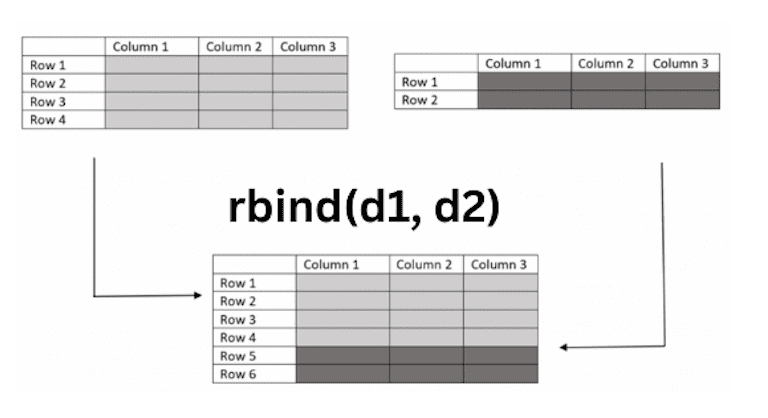# What is the rbind() Function in R

The rbind() function in R is “used to combine vectors, matrices, and data frames by rows.

## Syntax

``rbind(input_data, data_to_bind)``

## Parameters

1. input_data: It is input data.
2. data_to_bind: It is the data that needs to be bound.

## Visual representation## Example 1: Rbind vectors into a matrix

You can use the rbind() function in R to “combine vectors into a matrix by rows”.

``````# Create two vectors
a <- c(11, 31, 32, 41, 51)
b <- c(71, 72, 81, 31, 21)

# Rbind the two vectors into a matrix
rv_matrix <- rbind(a, b)

# View matrix
print(rv_matrix)
``````

Output

``````   [,1] [,2] [,3] [,4] [,5]
a   11   31   32   41   51
b   71   72   81   31   21
``````

## Example 2: Rbind Vector to a Data Frame

You can row-bind a single vector to a data frame using the “rbind()” function.

``````# Create a data frame
df <- data.frame(col1 = c("a", "b", "c"), col2 = c("A", "B", "C"))

# Create a vector
new_row <- c("new1", "new2")

# Append the vector as a new row to the data frame
df_new <- rbind(df, new_row)

# View the updated data frame
df_new
``````

Output

``````   col1 col2
1   a    A
2   b    B
3   c    C
4 new1 new2
``````

## Example 3: Rbind multiple vectors to a Data Frame

Use the “rbind()” function to row-bind multiple vectors to an existing data frame.

``````df <- data.frame(col1 = c("a", "b", "c"), col2 = c("A", "B", "C"))

# Create vectors
new_row1 <- c("new1", "new2")
new_row2 <- c("new3", "new4")

# Append the vectors as new rows to the data frame
df_new <- rbind(df, new_row1, new_row2)

# View the updated data frame
df_new
``````

Output

``````  col1   col2
1  a      A
2  b      B
3  c      C
4  new1  new2
5  new3  new4
``````

## Example 4: Rbind Two Data Frames

You can use the “rbind()” function to row-bind “two data frames”.

``````# Create two data frames
df1 <- data.frame(col1 = c("a", "b", "c"), col2 = c("A", "B", "C"))
df2 <- data.frame(col1 = c("d", "e", "f"), col2 = c("D", "E", "F"))

# Row-bind the two data frames
df_new <- rbind(df1, df2)

# View the updated data frame
df_new
``````

Output

``````  col1   col2
1  a      A
2  b      B
3  c      C
4  d      D
5  e      E
6  f      F
``````

That’s it!